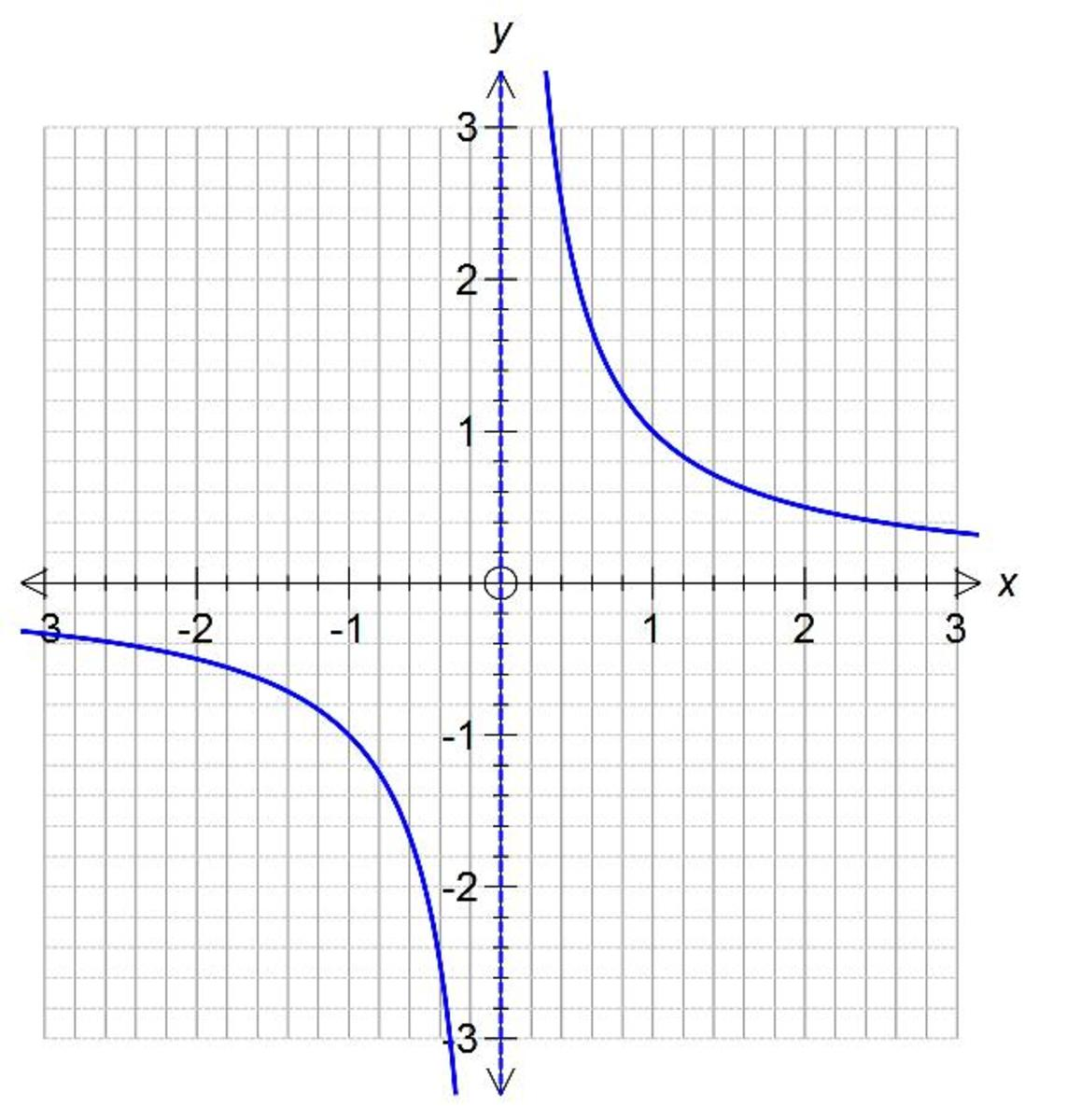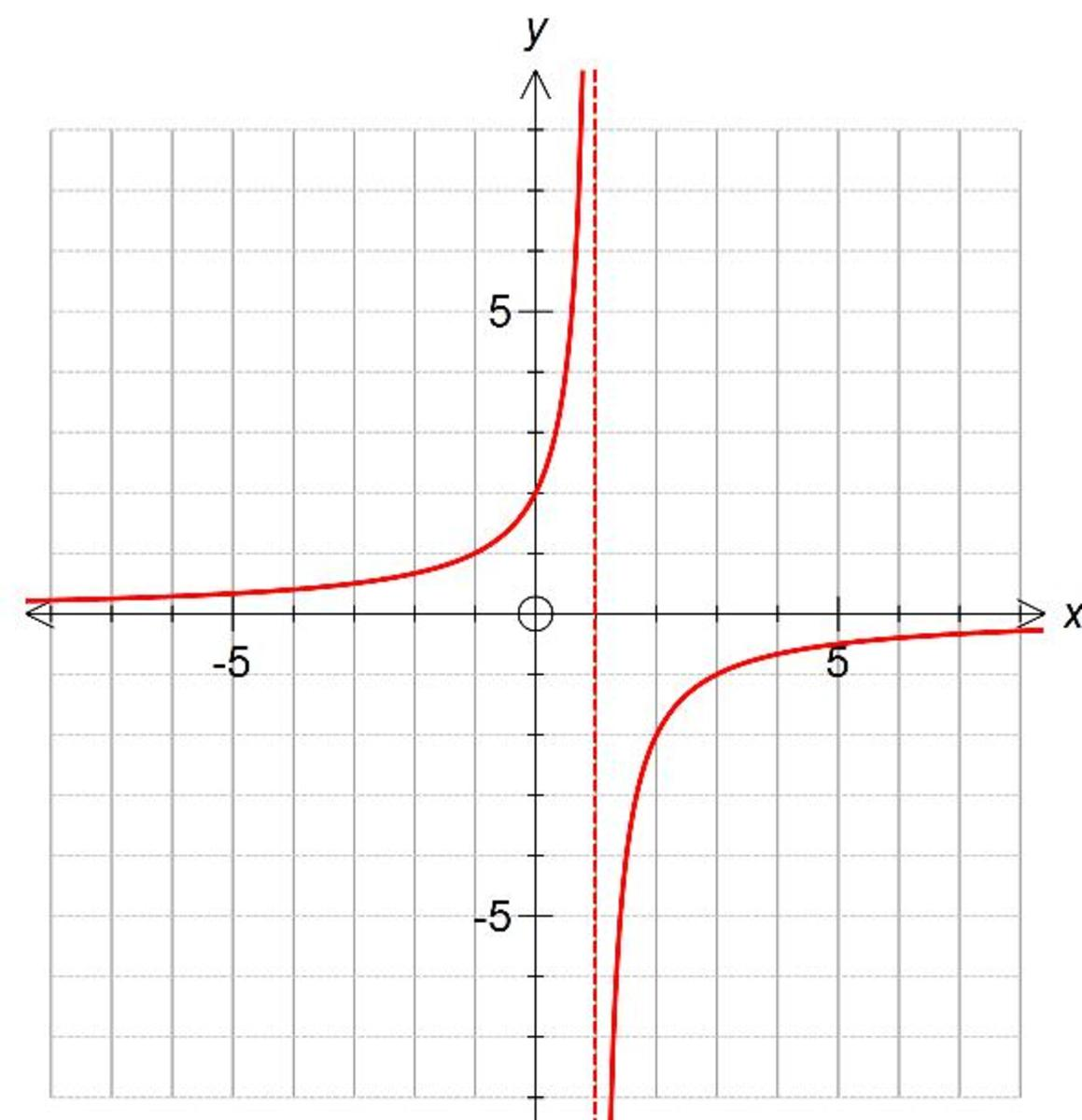Updated date:

# Reciprocal Graphs. What does a reciprocal graph look like and it's characteristics?

Author:In this Hub I will show you what a reciprocal graph looks like. The most simple types of reciprocal graphs take the form of y = A/x where A can be a positive or negative value.

Let’s take a look at a couple of examples of reciprocal graphs and then summarise the main characteristics at the end of this Hubpage.

Example 1

Plot the graph of y = 1/x from x = -3 to x = 3.

Let’s substitute the values into y =1/x and work out the y-coordinates to get an idea what is going on with this graph.

At x = -3, y = 1/-3 = -0.33

At x = -2, y = 1/-2 = -0.50

At x = -1, y = 1/-1 = -1.00

At x = 0, y = 1/0 = infinity

At x = 1, y = 1/1 = 1.00

At x = 2, y = ½ = 0.50

At x = 3, y = 1/3 = 1.33

What’s interesting about this reciprocal graph is that as x approaches 0 from the negative direction y approaches negative infinity. Also, as x approaches 0 from the positive direction y approaches positive infinity. Therefore, the graph has an asymptote at x = 0 (an asymptote is a line that a graph converges to).

Also the graph has an asymptote at y = 0 (the x axis) as the graph converges to these values as x get bigger in the positive and negative directions.Example 2

Plot the reciprocal graph of y = -2/(x-1). Plot the graph from x = -3 and x = 3.

Again let’s substitute integer values from x = -3 to x = 3.

At x = -3, y = -2/(-3-1) = ½ = 0.50

At x = -2, y = -2/(-2-1) = 0.67

At x = -1, y = -2/(-1-1) = 1.00

At x = 0, y = -2/(0-1) = 2.00

At x = 1, y = -2/(1-1) = infinity

At x = 2, y = -2/(2-1) = -2.00

At x =3, y = -2/(3-1) = -1.00

All you need to do is plot these points on the coordinate grid and drawn the graph (the graph shown goes from -8 to +8 to give you a better idea of what is going on).

If you notice this time that the graph converges to x =1 from the left and from the right. Therefore, the graph has a vertical asymptote at x = 1.

Also the graph has a horizontal asymptote at y = 0 (the x axis) as the graph converges to these values as x get bigger in the positive and negative directions.

So to summarise the main characteristics of a reciprocal graphs is that they are split into two parts and have a vertical asymptote and a horizontal asymptote.## Comments

cheddar on December 15, 2012:

1/0 does not equal infinity. It is undefined.

Patty Kenyon from Ledyard, Connecticut on July 13, 2012:

This is definitely useful information!!! Good Job!!!

Joan Whetzel on July 13, 2012:

Great explanation. Makes it easy to understand.